# Triacontagon

Regular triacontagon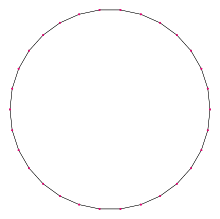A regular triacontagon
Type Regular polygon
Edges and vertices 30
Schläfli symbol {30}, t{15}
Coxeter diagram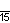Symmetry group Dihedral (D30), order 2×30
Internal angle (degrees) 168°
Dual polygon Self
Properties Convex, cyclic, equilateral, isogonal, isotoxal

In geometry, a triacontagon or 30-gon is a thirty-sided polygon. The sum of any triacontagon's interior angles is 5040 degrees.

## Regular triacontagon

The regular triacontagon is a constructible polygon, by an edge-bisection of a regular pentadecagon, and can also be constructed as a truncated pentadecagon, t{15}. A truncated triacontagon, t{30}, is a hexacontagon, {60}.

One interior angle in a regular triacontagon is 168°, meaning that one exterior angle would be 12°. The triacontagon is the largest regular polygon whose interior angle is the sum of the interior angles of smaller polygons: 168° is the sum of the interior angles of the equilateral triangle (60°) and the regular pentagon (108°).

The area of a regular triacontagon is (with t = edge length)The inradius of a regular triacontagon isThe circumradius of a regular triacontagon is### Construction

As 30 = 2 × 3 × 5, a regular triacontagon is constructible using a compass and straightedge.

## Symmetry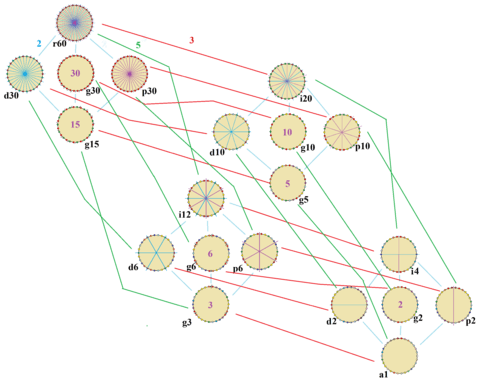The symmetries of a regular triacontagon as shown with colors on edges and vertices. Lines of reflections are blue through vertices, and purple through edges. Gyrations are given as numbers in the center. Vertices are colored by their symmetry positions.

The regular triacontagon has Dih30 dihedral symmetry, order 60, represented by 30 lines of reflection. Dih30 has 7 dihedral subgroups: Dih15, (Dih10, Dih5), (Dih6, Dih3), and (Dih2, Dih1). It also has eight more cyclic symmetries as subgroups: (Z30, Z15), (Z10, Z5), (Z6, Z3), and (Z2, Z1), with Zn representing π/n radian rotational symmetry.

John Conway labels these lower symmetries with a letter and order of the symmetry follows the letter. He gives d (diagonal) with mirror lines through vertices, p with mirror lines through edges (perpendicular), i with mirror lines through both vertices and edges, and g for rotational symmetry. a1 labels no symmetry.

These lower symmetries allows degrees of freedoms in defining irregular triacontagons. Only the g30 subgroup has no degrees of freedom but can seen as directed edges.

## Triacontagram

A triacontagram is a 30-sided star polygon. There are 3 regular forms given by Schläfli symbols {30/7}, {30/11}, and {30/13}, and 11 compound star figures with the same vertex configuration.

There are also isogonal triacontagrams constructed as deeper truncations of the regular pentadecagon {15} and pentadecagram {15/7}, and inverted pentadecagrams {15/11}, and {15/13}. Other truncations form double coverings: t{15/14}={30/14}=2{15/7}, t{15/8}={30/8}=2{15/4}, t{15/4}={30/4}=2{15/4}, and t{15/2}={30/2}=2{15}.

## Petrie polygons

The regular triacontagon is the Petrie polygon for three 8-dimensional polytopes with E8 symmetry, shown in orthogonal projections in the E8 Coxeter plane. It is also the Petrie polygon for two 4-dimensional polytopes, shown in the H4 Coxeter plane.

E8 H4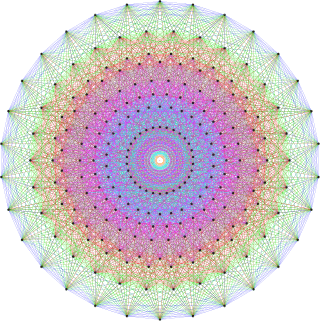421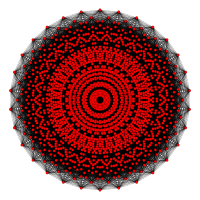241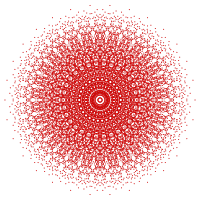142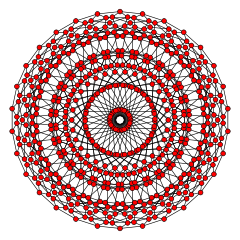120-cell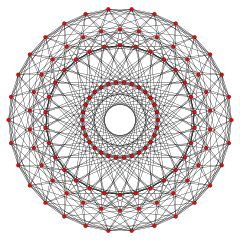600-cell

The regular triacontagram {30/7} is also the Petrie polygon for the great grand stellated 120-cell and grand 600-cell.

1. Constructible Polygon
2. The Symmetries of Things, Chapter 20
3. The Lighter Side of Mathematics: Proceedings of the Eugène Strens Memorial Conference on Recreational Mathematics and its History, (1994), Metamorphoses of polygons, Branko Grünbaum